Trisectrix of Maclaurin
TRISECTRIX OF MACLAURIN

Balmoral Software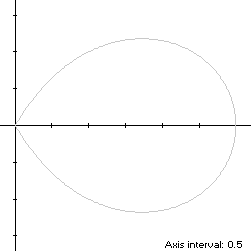The lobe of the Trisectrix of Maclaurin is a convex x-symmetric droplet-shaped closed curve S with polar equation
r(t) = 4 cos(t) - sec(t), -π/3 ≤ t < π/3
The curve follows a counterclockwise path as t increases, starting from the cusp at the origin when t = -π/3, proceeding through Quadrant IV to the right edge (3,0) at t = 0, then following a symmetric path through Quadrant I back to the cusp at t = π/3. The maximum ordinate point of S is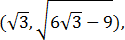so the width x height of its bounding rectangle is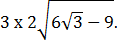### Metrics

The area of S is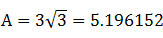and its perimeter is 8.244653. By (C2), its centroid abscissa is### Incircle

The maximum radius of a circle centered on the x-axis and inscribed in S is its maximum ordinate, so a candidate for the incircle has radius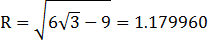and center abscissa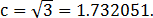The candidate circle must be contained within S, so we require that c - R and c + R both be within the abscissa range [0,3] of S, which is true. For verification, we have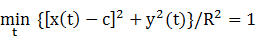### Inellipse

Using z = 3 in Lemma E,
d/dt [x(t) - z]y(t) = d/dt -4sin2(t)tan(t)[2cos(2t) + 1]
has a zero at t* = π/4. The corresponding coordinates are (x*,y*) = (1,1). We then have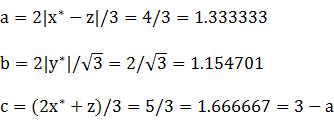For verification,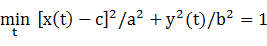### Circumellipse

Using z = 0 in Lemma E,
d/dt [x(t) - z]y(t) = d/dt [4cos(t) - sec(t)]2cos(t)sin(t)
has a zero at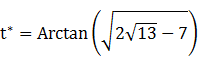The corresponding coordinates are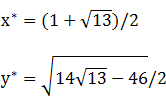We then have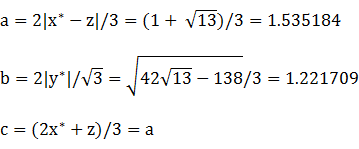For verification,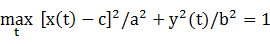### Circumcircle

Since the abscissa extrema are on the x-axis and the bounding rectangle is wider than it is tall, a candidate for the circumcircle has its radius and center abscissa both equal to 3/2. For verification, we have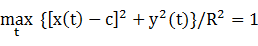### Summary Table

 Perimeter Area Centroid Figure Parameters Incircle R =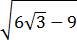7.413905 4.374055 (1.732051,0) Inellipse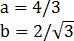7.826465 4.836799 (1.666667,0) Trisectrix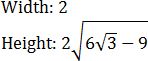8.244653 5.196152 (1.612266,0) Circumellipse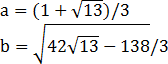8.689052 5.892208 (1.535184,0) Circumcircle R = 3/2 9.424778 7.068584 (1.5,0)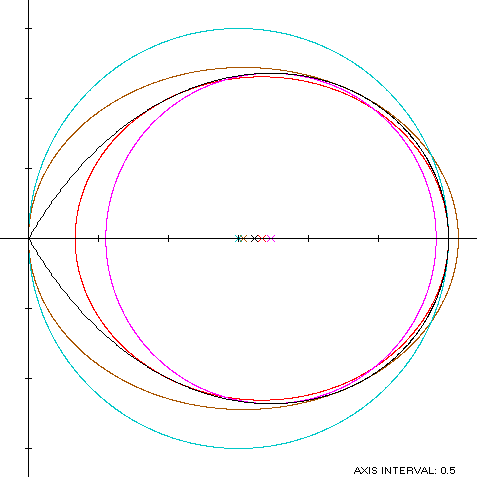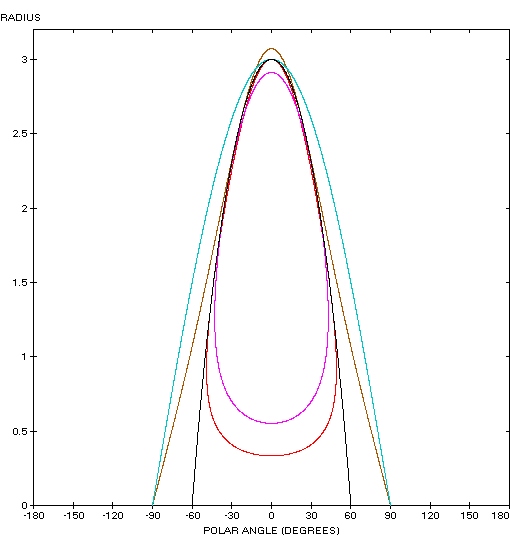The Trisectrix of Maclaurin (red) is a member of a group of similarly-shaped figures described on these pages, including (inside to outside) the teardrop curve, the Tschirnhausen cubic, the right strophoid and the piriform curve: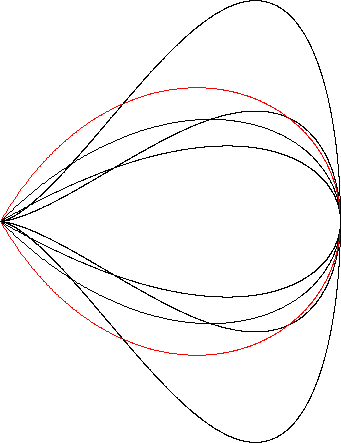### Relationship with the Folium of Descartes

The Folium of Descartes coincides with the Trisectrix of Maclaurin after a rotation and non-uniform scaling of the coordinates. The polar equation of the Folium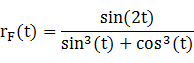is rotated clockwise by π/4, producing the new x-symmetric polar function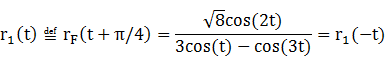Over the lobe domain -π/4 ≤ t < π/4, the maximum abscissa and ordinate of the rotated Folium are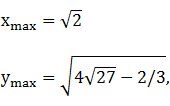so to scale the Folium to the comparable coordinate extrema of the trisectrix, we multiply its abscissas by 3/xmax and its ordinates by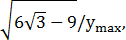producing the coordinate functions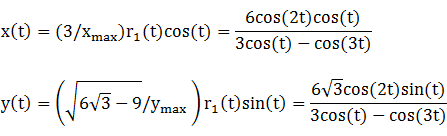These coordinate functions cannot be directly compared with the corresponding ones for the trisectrix since the rates of travel along the path (if t is considered a time variable) vary between the two curves, and the domains for t are different. However, the path itself is the same for both curves since the preceding x(t), y(t) satisfy the cartesian form of the trisectrix:
2x(x2 + y2) = 2(3x2 - y2)